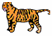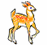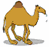Skill 9H Coordinate Graphs: Ordered Pairs: Points on a Grid Specific locations on graphs are identified by two numbers called an "ordered pair." This question uses pictures to test mastery of this skill. For example, the ordered pair 4,1 would be found on a grid by starting at 0,0 (bottom left corner) and then counting over 4 lines to the right and then 1 line up. So the tiger would be at the location 4,1.  So, the first number is moving horizontal while the second number is moving vertically.  Another helpful strategy is to have the student to learn the phrase - "over - up."This is a map of the Cypress Zoo. What is the ordered pairs for the location of each animal?1. What is the ordered pair for the? 2. What is the ordered pair for the? 3. What is the ordered pair for the? 4. What is the ordered pair for the? 5. What is the ordered pair for the?